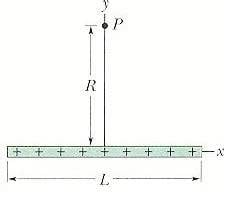# Find electric field produced at point P, along perp. bisector

## Homework Statement

In the figure below, positive charge q = 7.81 pC is spread uniformly along a thin nonconducting rod of length L = 12.5 cm.What is the magnitude of the electric field produced at point P, at distance R = 6.00 cm from the rod along its perpendicular bisector?

## Homework Equations

E= k*Q / r^2
dQ = lambda*dx
7.81 pC = 7.81E-12 C
lambda = Q / L

## The Attempt at a Solution

dQ = lambda*dx
dE = [k(lambda*dx) / (x^2 + R^2)] * R / (x^2 + R^2)^(1/2)

--> r^2 on the bottom = (x^2 + R^2) because it's the distance between dQ and point P
--> R / (x^2 + R^2)^(1/2) was found from cos theta between R and the line connecting points dQ and R
--> Since all the horizontal components cancel eachother out I only need to solve for the y-direction.
--> This is where I get confused is with the integral

Etotal = dEy = 2 [ integral (k(lambda*dx) / (x^2 + R^2)) * R / (x^2 + R^2)^(1/2)]

dEy = 2 [ integral (k*R(lambda*dx) / (x^2 + R^2)^(3/2))]
--> I'm integrating over .5L and 0, then multiplying the whole integral by 2 to make up for the other half of the rod. Now I'm just about completely lost by which terms to take out of the integral and which to leave in, heres my attempt:
dEy = 2*k*lambda*R [ integral (dx/ (x^2 + r^2) ^(3/2)) ]
Ey = 2*k*lambda*R*(2/5) [ 1 / (x^2 + R^2)^(5/2))
Ey = .312 which isn't right, any suggestions?

thanks